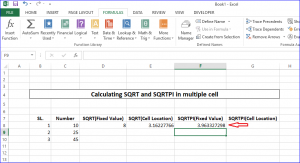# How to calculate Square Root and Square Root PI in Excel

One of the most useful feature of Excel is built-in excel function. We can perform different types of mathematical operations very easily in the worksheet by applying different excel functions as formula. The built-in functions of excel are used to calculate any general arithmetic operation and complex mathematical operation. Excel user don’t need to use calculator to find out any complicated calculation if they know how to apply the required function in the spreadsheet properly. One of the most important thing of using function is that the users can apply the same function in multiple cells where they require the calculation. Among the various excel functions, two common excel functions are SQRT and SQRTPI. SQRT function is used to find out the square root value of any number and SQRTPI function is used to find out the value of multiplying π with square root of any number. Both functions take a numeric value as parameter. How you can apply these functions in excel document is shown in this article.

Open an excel document to apply the use of SQRT and SQRTPI functions. Suppose, the following document is used to do the task. The use of these functions are shown here based on fixed value and particular cell value.Using SQRT function in Excel

Select the cell where you want to apply SQRT function and click on the formula bar. Type the following formula using SQRT function to calculate square root value of 64.

=SQRT(64)We know the value of √64 is 8. Press enter to activate the formula and you will get the result in the cell.If you want to apply this function based on cell value then write the formula using cell location.

=SQRT(c8)Press enter to show the result of the formula.Using SQRTPI function in Excel

Select a cell to apply SQRTPI function. Write the following formula using SQRTPI function with the value 5.

=SQRTPI(5)Press enter to activate the formula and show the value.We know that SQRTPI(number) = SQRT(number*π). So you can also do the above operation by writing the following formula in the next cell. Here, π value of 5 digits after decimal point is used to calculate.

=SQRT(5*3.14159)If you check the values after activating the formula then you will find that two values are similar. So you can use any one of the above formulas to calculate the square root π.You can apply SQRTPI function for any particular cell like SQRT function which is shown before.Now, if you want to use SQRT or SQRTPI function for multiple cells then you just select the cell where the function is added and place the mouse on bottom right corner of the cell. A + symbol will be appeared there. You need to drag the + symbol to the bottom for adding same formula function on other cells.The following value will appear after applying the formula on other cells. Using the same way you can copy any formula in other cell.